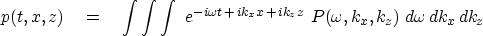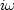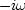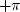Next: Examples of 2-D FT Up: TWO-DIMENSIONAL FT Previous: Basics of two-dimensional Fourier

## Signs in Fourier transforms

In Fourier transforming t-, x-, and z-coordinates, we must choose a sign convention for each coordinate. Of the two alternative sign conventions, electrical engineers have chosen one and physicists another. While both have good reasons for their choices, our circumstances more closely resemble those of physicists, so we will use their convention. For the inverse Fourier transform, our choice is(19)
For the forward Fourier transform, the space variables carry a negative sign, and time carries a positive sign.

Let us see the reasons why electrical engineers have made the opposite choice, and why we go with the physicists. Essentially, engineers transform only the time axis, whereas physicists transform both time and space axes. Both are simplifying their lives by their choice of sign convention, but physicists complicate their time axis in order to simplify their many space axes. The engineering choice minimizes the number of minus signs associated with the time axis, because for engineers, d/dt is associated withinstead of, as is the case for us and for physicists, with.We confirm this with equation (19). Physicists and geophysicists deal with many more independent variables than time. Besides the obvious three space axes are their mutual combinations, such as midpoint and offset.

You might ask, why not make all the signs positive in equation (19)? The reason is that in that case waves would not move in a positive direction along the space axes. This would be especially unnatural when the space axis was a radius. Atoms, like geophysical sources, always radiate from a point to infinity, not the other way around. Thus, in equation (19) the sign of the spatial frequencies must be opposite that of the temporal frequency.

The only good reason I know to choose the engineering convention is that we might compute with an array processor built and microcoded by engineers. Conflict of sign convention is not a problem for the programs that transform complex-valued time functions to complex-valued frequency functions, because there the sign convention is under the user's control. But sign conflict does make a difference when we use any program that converts real-time functions to complex frequency functions. The way to live in both worlds is to imagine that the frequencies produced by such a program do not range from toas the program description says, but from to.Alternately, we could always take the complex conjugate of the transform, which would swap the sign of the-axis.Next: Examples of 2-D FT Up: TWO-DIMENSIONAL FT Previous: Basics of two-dimensional Fourier
Stanford Exploration Project
10/21/1998